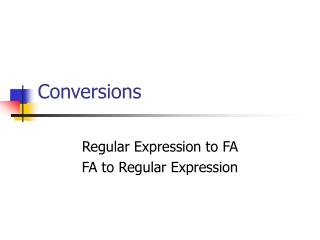DownloadDownload PresentationConversions

# Conversions

Télécharger la présentation## Conversions

- - - - - - - - - - - - - - - - - - - - - - - - - - - E N D - - - - - - - - - - - - - - - - - - - - - - - - - - -
##### Presentation Transcript

1. Conversions Regular Expression to FA FA to Regular Expression

2. Language Representing Regular Expressions • We define a mapping from a regular expression  to a language L() as follows: • Step 0: • L() = • L(e) = • L() = for each    • Inductive step: • L((  )) = • L(()) = • L(*) = {} {e} {} L()  L() L()L() L()*

3. Regular Languages Definition. A language is regular if there exists a regular expression that describes all its strings

4. Examples Example 1. Find L((ab*)a) L(ab*a) = L(a)L(b)*L(a) = {w : w is of the form abna with n = 0, 1, 2,…} Example 2. Find L((a(a b)*)). L(a(a b)*) = L(a)(L(a)  L(b))* = {aw : w is a word in }

5. Example Find the regular expression for the language L in the alphabet {a,b} such that the words in L contains the substring aaa Find the regular expression for the language L in the alphabet {a,b} such that the words in L contains the substring aaa or bbb

6. Main Theorem About Finite Automata (Kleene) (1) Given a finite automata A, there is a regular expression expr such that L(A) = L(expr) (2) Given a regular expression expr, there is a finite automata A such that L(A) = L(expr)

7. Algorithm A (from text) Input: a regular expression expr Output: a finite automaton accepting L(expr) • Convert any step-0 element (any of the characters in ,  or e) occurring in expr to a finite automaton accepting this element • Apply the theorem about closure under set operations to every • Union • Concatenation • Kleene star

8. Recall: Construction on NFA-ls l l M(R1) l l M(R) M(R2) l l M(R*) M(R1 U R2) l l l M(R1) M(R2) M(R1 R2)

9. Exercise • Obtain a finite automaton accepting a regular expression: • One or two in class • How about: • (a  a*b) a (ba*) • (a* b*) a*b+a • Make up several! • Use the union, concatenation, and * operations

10. Algorithm B (from text) Input: a finite automaton A Output: a regular expression expr such that L(expr) = L(A) • Assumptions about the automaton A: • A has a single favorable state • If it doesn’t, can this be taken care of ? • There are no transitions directed to the initial state • If there is one, can this be taken care of ? • There are no transitions starting at the favorable state Definition. An expression diagram is a labeled directed graph like a FSA, but with transitions labeled by regular expressions. as opposed to single elements of .

11. Algorithm B – States to RE Unions For every pair of nodes such that there is more than one transition from one to the other one: expr1 expr2 … exprn expr1 expr2 … exprn

12. expr1 (expr2)* expr3 Algorithm B – States to RE Kleene * • For every pair of nodes such that there is an intermediate node connecting them: expr3 expr1 expr2

13. b q0 q1 a a q2 b Simple Example

14. q0 a q2 b Delete node q1 b q1 a ab

15. q0 Delete node q2 (ab*ab)* a ab q2 b

16. b q0 q1 a b a b a q2 q3 b Detailed Example

17. b q0 q1 a bb b a ab b a q2 q3 b Delete node q1

18. q0 b bb a a q2 q3 b Delete node q2 ab*ab ab

19. q0 b bb a q3 Finally ab*ab (ab*ab)*a ((b bb) (ab*ab)*a)*